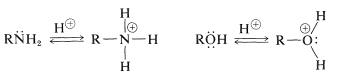# 23.1: Amines Compared with Alcohols

As you read the chapter you will realize a similarity between the chemistry of amines and the chemistry of alcohols, which we discussed in Chapter 15. Primary amines $$\left( \ce{RNH_2} \right)$$ and secondary amines $$\left( \ce{R_2NH} \right)$$ are much weaker acids than alcohols $$\left( \ce{ROH} \right)$$ and form strongly basic anions:

AcidsAmines, like alcohols, have nonbonding electrons that impart basic and nucleophilic properties:

BasesNucleophilesAlso, amines and alcohols both can behave as carbon electrophiles under appropriate reaction conditions such that cleavage of $$\ce{C-N}$$ and $$\ce{C-O}$$ bonds occurs in the sense $$\overset{\delta \oplus}{\ce{C}} \vdots \overset{\delta \ominus}{\ce{N}}$$ and $$\overset{\delta \oplus}{\ce{C}} \vdots \overset{\delta \ominus}{\ce{O}}$$. However, because $$\ce{-NH_2}$$ and $$\ce{-OH}$$ both are poor leaving groups, each must be suitably activated to make this kind of reaction possible (see Section 8-7C). The $$\ce{OH}$$ group can be activated by addition of a proton or conversion to a sulfonate ester, $$\ce{RO_3SR'}$$, but these processes generally are ineffective for $$\ce{RNH_2}$$. The most effective activation for $$\ce{RNH_2}$$ is through conversion with nitrous acid, $$\ce{HONO}$$, to $$\ce{R}- \overset{\oplus}{\ce{N}} \equiv \ce{N}$$; then $$\ce{N_2}$$ is the leaving group (this reaction is described in more detail in Section 23-10A):

$\ce{R-OH} \overset{\ce{HBr}}{\longrightarrow} \ce{R-Br} + \ce{H_2O}$

There is, though a major difference in the way that amines and alcohols behave toward oxidizing agents. Amines genearlly show more complex behavior on oxidation because, as we shall see, nitrogen has a larger number of stable oxidation states than oxygen.